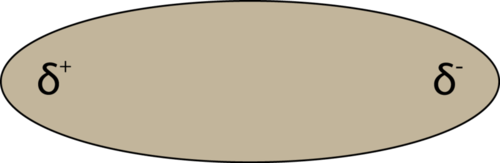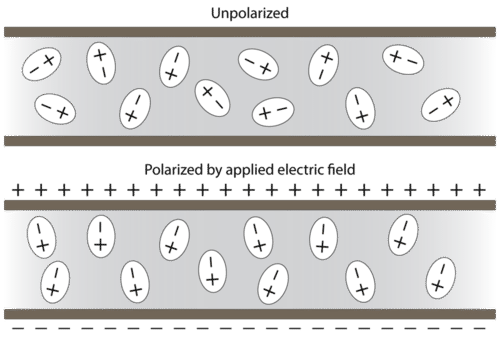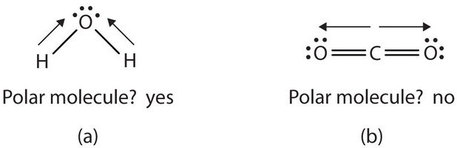# 4.4: Characteristics of Covalent Bonds

•• Contributed by Anonymous
• LibreTexts

Learning Objectives

• Recognize bond characteristics of covalent compounds: bond length and bond polarity.
• Use electronegativity values to predict bond polarity.

## Bond Length

We previously stated that the covalent bond in the hydrogen molecule (H2) has a certain length (about 7.4 × 10−11 m). Other covalent bonds also have known bond lengths, which are dependent on both the identities of the atoms in the bond and whether the bonds are single, double, or triple bonds. Table $$\PageIndex{1}$$ lists the approximate bond lengths for some single covalent bonds. The exact bond length may vary depending on the identity of the molecule but will be close to the value given in the table.

Table $$\PageIndex{1}$$: Approximate Bond Lengths of Some Single Bonds
Bond Length (× $$10^{−12}\, m$$)
H–H 74
H–C 110
H–N 100
H–O 97
H–I 161
C–C 154
C–N 147
C–O 143
N–N 145
O–O 145

Table $$\PageIndex{2}$$ compares the lengths of single covalent bonds with those of double and triple bonds between the same atoms. Without exception, as the number of covalent bonds between two atoms increases, the bond length decreases. With more electrons between the two nuclei, the nuclei can get closer together before the internuclear repulsion is strong enough to balance the attraction.

Table $$\PageIndex{2}$$: Comparison of Bond Lengths for Single and Multiple Bonds
Bond Length (× $$10^{−12}\, m$$)
C–C 154
C=C 134
C≡C 120
C–N 147
C=N 128
C≡N 116
C–O 143
C=O 120
C≡O 113
N–N 145
N=N 123
N≡N 110
O–O 145
O=O 121

## Electronegativity and Bond Polarity

Although we defined covalent bonding as electron sharing, the electrons in a covalent bond are not always shared equally by the two bonded atoms. Unless the bond connects two atoms of the same element, as in H2, there will always be one atom that attracts the electrons in the bond more strongly than the other atom does, as in HCl, shown in Figure $$\PageIndex{1}$$. A covalent bond that has an equal sharing of electrons (Figure $$\PageIndex{1a}$$) is called a nonpolar covalent bond. A covalent bond that has an unequal sharing of electrons, as in Figure $$\PageIndex{1b}$$, is called a polar covalent bond.Figure $$\PageIndex{1}$$ Polar versus Nonpolar Covalent Bonds. (a) The electrons in the covalent bond are equally shared by both hydrogen atoms. This is a nonpolar covalent bond. (b) The chlorine atom attracts the electrons in the bond more than the hydrogen atom does, leading to an imbalance in the electron distribution. This is a polar covalent bond.

The distribution of electron density in a polar bond is uneven. It is greater around the atom that attracts the electrons more than the other. For example, the electrons in the H–Cl bond of a hydrogen chloride molecule spend more time near the chlorine atom than near the hydrogen atom. Note that the shaded area around Cl in Figure $$\PageIndex{1b}$$ is much larger than it is around H.

This imbalance in electron density results in a buildup of partial negative charge (designated as δ−) on one side of the bond (Cl) and a partial positive charge (designated δ+) on the other side of the bond (H). This is seen in Figure $$\PageIndex{2a}$$. The separation of charge in a polar covalent bond results in an electric dipole (two poles), represented by the arrow in Figure $$\PageIndex{2b}$$. The direction of the arrow is pointed toward the δ− end while the + tail of the arrow indicates the δ+ end of the bond.Figure $$\PageIndex{2}$$: (a) Unequal sharing of the bonding pair of electrons between H and Cl leads to partial positive charge on the H atom and partial negative charge on the Cl. Symbols δ+ and δ– indicate the polarity of the H–Cl bond. (b) The dipole is represented by an arrow with a cross at the tail. The cross is near the δ+ end and the arrowhead coincides with the δ–.

Any covalent bond between atoms of different elements is a polar bond, but the degree of polarity varies widely. Some bonds between different elements are only minimally polar, while others are strongly polar. Ionic bonds can be considered the ultimate in polarity, with electrons being transferred rather than shared. To judge the relative polarity of a covalent bond, chemists use electronegativity, which is a relative measure of how strongly an atom attracts electrons when it forms a covalent bond. There are various numerical scales for rating electronegativity. Figure $$\PageIndex{3}$$ shows one of the most popular—the Pauling scale.

Looking Closer: Linus Pauling

Arguably the most influential chemist of the 20th century, Linus Pauling (1901–94) is the only person to have won two individual (that is, unshared) Nobel Prizes. In the 1930s, Pauling used new mathematical theories to enunciate some fundamental principles of the chemical bond. His 1939 book The Nature of the Chemical Bond is one of the most significant books ever published in chemistry.

By 1935, Pauling’s interest turned to biological molecules, and he was awarded the 1954 Nobel Prize in Chemistry for his work on protein structure. (He was very close to discovering the double helix structure of DNA when James Watson and James Crick announced their own discovery of its structure in 1953.) He was later awarded the 1962 Nobel Peace Prize for his efforts to ban the testing of nuclear weapons.Linus Pauling was one of the most influential chemists of the 20th century.

In his later years, Pauling became convinced that large doses of vitamin C would prevent disease, including the common cold. Most clinical research failed to show a connection, but Pauling continued to take large doses daily. He died in 1994, having spent a lifetime establishing a scientific legacy that few will ever equal.

The polarity of a covalent bond can be judged by determining the difference in the electronegativities of the two atoms making the bond. The greater the difference in electronegativities, the greater the imbalance of electron sharing in the bond. Although there are no hard and fast rules, the general rule is if the difference in electronegativities is less than about 0.4, the bond is considered nonpolar; if the difference is greater than 0.4, the bond is considered polar. If the difference in electronegativities is large enough (generally greater than about 1.8), the resulting compound is considered ionic rather than covalent. An electronegativity difference of zero, of course, indicates a nonpolar covalent bond.Figure $$\PageIndex{4}$$: As the electronegativity difference increases between two atoms, the bond becomes more ionic.

Example $$\PageIndex{1}$$

Describe the electronegativity difference between each pair of atoms and the resulting polarity (or bond type).

1. C and H
2. H and H
3. Na and Cl
4. O and H

Solution

1. Carbon has an electronegativity of 2.5, while the value for hydrogen is 2.1. The difference is 0.4, which is rather small. The C–H bond is therefore considered nonpolar.
2. Both hydrogen atoms have the same electronegativity value—2.1. The difference is zero, so the bond is nonpolar.
3. Sodium’s electronegativity is 0.9, while chlorine’s is 3.0. The difference is 2.1, which is rather high, and so sodium and chlorine form an ionic compound.
4. With 2.1 for hydrogen and 3.5 for oxygen, the electronegativity difference is 1.4. We would expect a very polar bond. The sharing of electrons between O and H is unequal with the electrons more strongly drawn towards O.

Exercise $$\PageIndex{1}$$

Describe the electronegativity (EN) difference between each pair of atoms and the resulting polarity (or bond type).

1. C and O
2. K and Br
3. N and N
4. Cs and F

The EN difference is 1.0 , hence polar. The sharing of electrons between C and O is unequal with the electrons more strongly drawn towards O.

The EN difference is greater than 1.8, hence ionic.

Identical atoms have zero EN difference, hence nonpolar.

The EN difference is greater than 1.8, hence ionic.

## Bond Polarity and Molecular Polarity

If there is only one bond in the molecule, the bond polarity determines the molecular polarity. Any diatomic molecule in which the two atoms are the same element must be a nonpolar molecule. A diatomic molecule that consists of a polar covalent bond, such as HF, is a polar molecule. A polar molecule is a molecule in which one end of the molecule is slightly positive, while the other end is slightly negative. The two electrically charged regions on either end of the molecule are called poles, similar to a magnet having a north and a south pole. Hence, a molecule with two poles is called a dipole. A simplified way to depict polar molecules like HF is pictured below (see figure below).Figure $$\PageIndex{5}$$: A molecular dipole results from the unequal distribution of electron density throughout a molecule.

When placed between oppositely charged plates, polar molecules orient themselves so that their positive ends are closer to the negative plate and their negative ends are closer to the positive plate (see Figure 4.4.6 below).Figure $$\PageIndex{6}$$: Polar molecules are randomly oriented in the absence of an applied electric field (top). In an electric field, the molecules orient themselves to maximize the attraction between opposite charges (bottom).

Experimental techniques involving electric fields can be used to determine if a certain substance is composed of polar molecules and to measure the degree of polarity.

For molecules with more than two atoms, the molecular geometry must also be taken into account when determining if the molecule is polar or nonpolar. (Figure $$\PageIndex{7}$$) is a comparison between carbon dioxide and water. Carbon dioxide (CO2) is a linear molecule. The oxygen atoms are more electronegative than the carbon atom, so there are two individual dipoles pointing outward from the C atom to each O atom. However, since the dipoles are of equal strength and are oriented directly opposite each other, they cancel each other out, and the overall molecular polarity of CO2 is zero. CO2 is a nonpolar molecule. In H2O, the orientation of the two O–H bonds is bent: one end of the molecule has a partial positive charge, and the other end has a partial negative charge. In short, the molecule itself is polar. The polarity of water has an enormous impact on its physical and chemical properties. For example, the boiling point of water (100°C) is high for such a small molecule due to the fact that polar molecules attract each other strongly. On the other hand, the nonpolar carbon dioxide becomes a gas at −77°C, almost 200° lower than the temperature at which water boils. More details will be presented in the next section.Figure $$\PageIndex{7}$$ Physical Properties and Polarity. The physical properties of water and carbon dioxide are affected by their polarities.

## Concept Review Exercises

1. What is the name for the distance between two atoms in a covalent bond?

2. What does the electronegativity of an atom indicate?

3. What type of bond is formed between two atoms if the difference in electronegativities is small? Medium? Large?

1. bond length

2. Electronegativity is a qualitative measure of how much an atom attracts electrons in a covalent bond.

3. nonpolar; polar; ionic

## Key Takeaways

• Covalent bonds between different atoms have different bond lengths.
• Covalent bonds can be polar or nonpolar, depending on the electronegativity difference between the atoms involved.

## Exercises

1. Which is longer—a C–H bond or a C–O bond? (Refer to Table $$\PageIndex{1}$$.)

2. Which is shorter—an N–H bond or a C–H bond? (Refer to Table $$\PageIndex{1}$$.)

3. A nanometer is 10−9 m. Using the data in Table $$\PageIndex{1}$$ and Table $$\PageIndex{2}$$, determine the length of each bond in nanometers.

1. a C–O bond
2. a C=O bond
3. an H–N bond
4. a C≡N bond
4. An angstrom (Å) is defined as 10−10 m. Using Table $$\PageIndex{1}$$ and Table $$\PageIndex{2}$$, determine the length of each bond in angstroms.

1. a C–C bond
2. a C=C bond
3. an N≡N bond
4. an H–O bond
5. Refer to Exercise 3. Why is the nanometer unit useful as a unit for expressing bond lengths?

6. Refer to Exercise 4. Why is the angstrom unit useful as a unit for expressing bond lengths?

7. Using Figure $$\PageIndex{3}$$, determine which atom in each pair has the higher electronegativity.

1. H or C
2. O or Br
3. Na or Rb
4. I or Cl
8. Using Figure $$\PageIndex{3}$$, determine which atom in each pair has the lower electronegativity.

1. Mg or O
2. S or F
3. Al or Ga
4. O or I
9. Will the electrons be shared equally or unequally across each covalent bond? If unequally, to which atom are the electrons more strongly drawn?

1. a C–O bond
2. an F–F bond
3. an S–N bond
4. an I–Cl bond

10. Will the electrons be shared equally or unequally across each covalent bond? If unequally, to which atom are the electrons more strongly drawn?

• a C–C bond
• a S–Cl bond
• an O–H bond
• an H–H bond

11. Arrange the following bonds from least polar to most polar: H-F, H-N, H-O, H-C

12. Arrange the following bonds from least polar to most polar: C-F, C-N, C-O, C-C

1. A C–O bond is longer.

2. An H-N bond is shorter than an H-C bond.
1. 0.143 nm
2. 0.120 nm
3. 0.100 nm
4. 0.116 nm

4.

1. 1.54 Å
2. 1.34 Å
3. 1.10 Å
4. 0.97 Å
1. Actual bond lengths are very small, so the nanometer unit makes the expression of length easier to understand.

6. Actual bond lengths are very small, so the angstrom unit makes the expression of length easier to understand.
1. C
2. O
3. Na
4. Cl
8.
1. Mg
2. S
3. Al
4. I

9.

1. unequally toward the O
2. equally
3. unequally toward the N
4. unequally toward the Cl

10.

• equally
• unequally toward the Cl
• unequally toward the O
• equally

11. The electronegativity difference increases from 0.4; 0.9; 1.4; 1.9. Hence, the least to most polar: H-C, H-N, H-O, H-F

12. The electronegativity difference increases from 0; 0.5; 1.0; 1.5. Hence, the least to most polar: C-C, C-N, C-O, C-F